Definitions of Square Dance Calls and Concepts

Index -->  Plus  |  A1  |  A2  |  C1  |  C2  |  C3A  |  C3B  |  C4  |  NOL  |
Definitions (Text Only) -->  Plus  |  A1  |  A2  |  C1  |  C2  |  C3A  |  C3B  |  C4  |  NOL  |
 Find call:

 \$B8@8l(BMini Wave \$B\$N(B Box (\$B\$^\$?\$O(B fraction \$B\$,\$"\$k\$H\$-\$O(B, \$BB>\$NE,@Z\$J(B 2 x 2) \$B\$+\$i(B.

1. 1/2 Zoom;
2. Hinge;
3. 1/2 Zoom;
4. Hinge.

Mini Wave \$B\$N(B Box \$B\$G=*\$o\$j\$^\$9(B. Reset \$B\$O(B 4 \$B%Q!<%H\$N%3!<%k\$G\$9(B.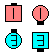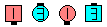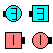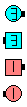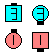Reset\$B\$NA0(B 1/2 Zoom(1/4)\$B\$N8e(B Hinge(1/2)\$B\$N8e(B 1/2 Zoom(3/4)\$B\$N8e(B Hinge\$B\$N8e(B (\$B=*\$o\$j(B)

\$BCm
• Reset \$B\$K\$O(B, \$B\$7\$P\$7\$P(B fraction \$B\$,IU\$1\$i\$l\$^\$9(B.
• Phantom \$B\$HMY\$k\$H\$-\$O(B, Reset \$B\$O(B Box Circulate Twice \$B\$HF1\$8F0\$-\$K\$J\$k\$3\$H\$rCN\$C\$F\$\$\$k\$H(B, \$B=u\$1\$K\$J\$j\$^\$9(B.
• Cross Reset [C4]: Mini Wave \$B\$N(B Box \$B\$+\$i(B. 1/2 Cross Zoom (Leaders Trail Off, Trailers 1/2 Circulate); Hinge; 1/2 Cross Zoom; Hinge \$B\$r\$7\$^\$9(B. Mini Wave \$B\$N(B Box \$B\$G=*\$o\$j\$^\$9(B.Choreography for ResetComments? Questions? Suggestions?

https://www.ceder.net/def/reset.php?language=japan
28-May-2020 13:07:51